# The Navier-Stokes Equations

## Fluid Dynamics

Fluid dynamics is a field in science and engineering that describes the flow of fluids, i.e. liquids and gases, with many subfields such as aerodynamics and hydrodynamics. To solve problems in fluid dynamics, physical properties of the fluids such as velocity, pressure, density, and temperature are taken under consideration as functions of space and time. There are many practical applications of fluid dynamics including the calculation of forces and moments on aircraft, the flow of fluids through pipes and around bluff bodies, prediction of weather patterns, and understanding nebulae in interstellar space.

The foundational axioms of fluid dynamics are the conservation laws, in particular, the conservation of mass, conservation of linear momentum, and conservation of energy. In addition to these conservation laws, fluids are assumed to obey the continuum assumption. That is, in fluid dynamics the focus is not on the properties of a single particle but rather on a point in space (relative to some fixed coordinate system). As time proceeds different fluids flow past these points in space and a history of the point’s physical properties are kept rather than tracking the individual particles as they travel through space and time.

### Fluid Dynamics Definitions

There are many types of fluids that flow in different ways. Below are the definitions of some requisite terms to build a vocabulary necessary for discussing fluid flow.

#### Pressure

Pressure in a static fluid is defined as the normal compressive force per unit area acting on a surface immersed in the fluid. The pressure in a fluid at rest is isotropic, i.e. the force acts with equal magnitude in all directions (independent of the direction of measurement). In a dynamic situation, there may be other shear and stress forces but the pressure is still isotropic and defined in the same way as the static case. However, the pressure must be measured as the normal stress on an area that moves along locally with the fluid.

#### Compressible Flow

All fluids are compressible to some extent. However, the density of a gas changes much more readily with fluctuations in temperature and pressure than does the density of a liquid. Thus, for most intents and purposes a gas is considered compressible while liquids, whose change in density is often negligible, are considered incompressible. Sound propagation in liquids is one of the few situations in which the compressibility of liquids must be considered.

#### Newtonian Fluids

As shown by Isaac Newton, in common fluids, such as air and water, the stress due to viscous forces from neighboring parcels of fluids moving at different velocities is linearly related to the strain rate. Fluids obeying this linear relationship are called Newtonian fluids and the coefficient of proportionality is the fluid’s viscosity. Fluids with more complicated non-linear stress-strain behavior are called non-Newtonian fluids.

#### Inviscid, Viscous, and Stokes Flow

Stokes flow, or creeping flow, is fluid flow in which the viscous forces are very strong compared to the inertial forces. In such cases, the inertial forces are sometimes neglected. In contrast, when inertial forces have more effect on the velocity field than viscous (friction) forces the flow is often modeled as inviscid. Inviscid flow is an approximation in which viscosity is completely neglected. In cases involving solid boundaries, the no-slip condition generates a region of large strain rate (the boundary layer) in which viscosity effects dominate and vorticity is generated. In such cases, viscous flow equations, such as Navier-Stokes equations, must be used to calculate the net forces on the bodies.

A flow that does not depend on time is known as a steady flow. Contrarily, a time-dependent flow is known as unsteady or transient.

#### Laminar vs. Turbulent Flow

Laminar flow is a purely viscous flow in which the fluid flows in laminae or layers. A stream of dye inserted into a laminar flow will streak out into a thin line and always be composed of the same fluid particles. In contrast, the turbulent flow has velocity components with random turbulent fluctuations imposed upon their mean values. Dye inserted into such a flow would quickly become tangled and mixed in with the fluid as it flows along. Turbulence, which is caused by excessive kinetic energy in parts of fluid flow, is one of the least understood natural phenomena.

## The Navier-Stokes Equations

The Navier-Stokes equations are a set of nonlinear partial differential equations that describe fluid flow. The equations are an extension of Euler’s equations which can only be used to model inviscid fluid flow as there is no term to account for a fluid’s viscosity. The extension of Euler’s equations was presented by Claude-Louis Navier, a French mathematician and engineer, in 1822. Although his equations were correct, there were flaws in the reasoning of Navier’s mathematical argument. Later, in 1842, George Stokes, an Irish mathematician, began looking at problems in fluid dynamics and, among other things, provided a correct derivation of Navier’s equations thus giving them their name.

There are three sets of vector-valued equations that make up the Navier-Stokes equations that must be used to accurately model fluid flow, viz. equations for the conservation of mass (or continuity equation), the conservation of momentum, and the conservation of energy. However, in many cases, the conservation of energy equation can be neglected since many flow fields are assumed to be isothermal. Thusly, to model fluid flow one must solve the conservation of momentum equations with solutions restricted by the conservation of mass.

These equations are given by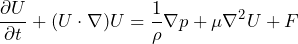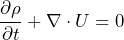The first equation here is the conservation of momentum and the second is the conservation of mass or continuity equation. The terms are defined as:

•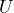– a vector valued variable representing the flow’s velocity components
•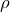– the fluid density, for incompressible flows this is constant
•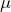– the fluid’s viscosity, again this is usually assumed to be constant
•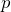– a vector valued variable/function that represents the pressure field of the flow
•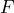– a vector valued variable which represents body forces

#### Conservation of Mass

If the fluid is incompressible, by definition, density is constant throughout the fluid, i.e.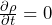. Therefore, the continiuity equation becomes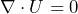The physical meaning of the left-hand side of the continuity equation, i.e. the divergence of velocity, is somewhat elusive due to its mathematical expansion, e.g. in three dimensions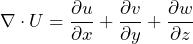However, put simply, the divergence of velocity models the change in volume of a fluid element as it moves through the flow field.

#### Conservation of Momentum

The conservation of momentum equation repeated below for convenience is really just Newton’s second law of motion in disguise.The equation models the forces acting on a fluid and equates them to the fluid’s mass times its acceleration. Term-by-term the momentum equation contains

•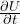– the unsteady term which models the change of velocity with respect to time
•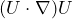– the convective acceleration term models how a particle of fluid’s velocity will change as it moves from place to place in the flow field
•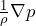– the gradient of the pressure field multiplied by 1 over its mass
•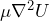– depends on the viscosity of the fluid and models viscous diffusion in the flow
•– a vector of values that represents the body forces acting on the fluid

Note that this is a particular expression of the Navier-Stokes equations. The form of the continuity equations and the momentum equations assume that we are modeling a Newtonian fluid and an incompressible flow. Additionally, since we are neglecting the energy equation the flow is assumed to be isothermal.

## Conclusion

My motivation for this post was to provide a brief introduction to the Navier-Stokes equations so that I can reference it in future writings about my master’s thesis and implementations of fluid flow solvers. Above I have provided a brief overview of some terms in fluid dynamics that are useful for understanding the Navier-Stokes equations and help to build a vocabulary for talking about fluid flows. Although I have never been formally taught fluid dynamics, my master’s thesis had a heavy emphasis on the field and I have spent a lot of time in self-study. So far, the equations and assumptions provided above have been used in all of my work in this area.

Again, I have never formally been taught fluid dynamics so if there are any mistakes in this post please call me out as to not spread misinformation.